Practice Test: Electronics Engineering (ECE)- 12

# Practice Test: Electronics Engineering (ECE)- 12 - Electronics and Communication Engineering (ECE)

Test Description

## 65 Questions MCQ Test GATE ECE (Electronics) 2024 Mock Test Series - Practice Test: Electronics Engineering (ECE)- 12

Practice Test: Electronics Engineering (ECE)- 12 for Electronics and Communication Engineering (ECE) 2023 is part of GATE ECE (Electronics) 2024 Mock Test Series preparation. The Practice Test: Electronics Engineering (ECE)- 12 questions and answers have been prepared according to the Electronics and Communication Engineering (ECE) exam syllabus.The Practice Test: Electronics Engineering (ECE)- 12 MCQs are made for Electronics and Communication Engineering (ECE) 2023 Exam. Find important definitions, questions, notes, meanings, examples, exercises, MCQs and online tests for Practice Test: Electronics Engineering (ECE)- 12 below.
Solutions of Practice Test: Electronics Engineering (ECE)- 12 questions in English are available as part of our GATE ECE (Electronics) 2024 Mock Test Series for Electronics and Communication Engineering (ECE) & Practice Test: Electronics Engineering (ECE)- 12 solutions in Hindi for GATE ECE (Electronics) 2024 Mock Test Series course. Download more important topics, notes, lectures and mock test series for Electronics and Communication Engineering (ECE) Exam by signing up for free. Attempt Practice Test: Electronics Engineering (ECE)- 12 | 65 questions in 180 minutes | Mock test for Electronics and Communication Engineering (ECE) preparation | Free important questions MCQ to study GATE ECE (Electronics) 2024 Mock Test Series for Electronics and Communication Engineering (ECE) Exam | Download free PDF with solutions
 1 Crore+ students have signed up on EduRev. Have you?
Practice Test: Electronics Engineering (ECE)- 12 - Question 1

### Which of the following states the meaning of the idiom ‘Into the bargain’?

Detailed Solution for Practice Test: Electronics Engineering (ECE)- 12 - Question 1
‘Into the bargain’ has the meaning‘ in addition to what has already been mentioned or was expected’
Practice Test: Electronics Engineering (ECE)- 12 - Question 2

### Direction: Find the correct relationship between the given quantities-Quantity I: Let E be the set of all integers with 6 in their unit place. The probability that a number chosen from the set {10, 11, 12, ........59} is a member of E is?Quantity II: A boy speaks truth in 70% of cases while another boy speaks truth in 65% cases. The probability that they will contradict each other on stating the same fact is?

Detailed Solution for Practice Test: Electronics Engineering (ECE)- 12 - Question 2
Quantity I: The sample space is n(s) = 50

The favourable outcomes are {16, 26, 36, 46, 56} i.e. 5

Probability = 5/50 = 0.1

Quantity II: Boy 1 speaks truth in 70% cases so 70/100 =7/10 and

Boy 2: 65/100 = 13/20

Boy 2 does not speak truth in 35/100 cases i.e. 7/20

Probability= p (A')*p (B) +p (A)*p (B’)

[7*7/ (10*20)]+ [3*13/ (10*20)]

= 0.44

Practice Test: Electronics Engineering (ECE)- 12 - Question 3

### A square metallic sheet of an area of 1296 square cm is used for making the toys. For taking the metallic sheet from that square – four equal sized of the circular plates have been cut –off. If a fencing with the Silver thread is required to make the base of toys, how many rolls of the thread is required, if a roll contains 30 cm of the thread only?

Detailed Solution for Practice Test: Electronics Engineering (ECE)- 12 - Question 3
Side * Side = 1296

⇒ Side= 36

Radius of each circular plate = ¼ of 36 = 9 cm

Circumference of each plate = 2 π 9 = 56.57 cm

Total Circumference = 4 *56.57 = 226.29 cm

No. of rolls = 226.29/30= 7.54 = 8 rolls

Practice Test: Electronics Engineering (ECE)- 12 - Question 4

The wrong (misfit) number of the sequence 36, 81, 144, 225, 256, 441 is

Detailed Solution for Practice Test: Electronics Engineering (ECE)- 12 - Question 4
The pattern in the above sequence is the squares of consecutive multiples of 3;

62 = 36

92 = 81

122 = 144

152 = 225

182 = 324 ≠ 256

212 = 441

Thus the wrong number is 256

Hence Option D is correct

Practice Test: Electronics Engineering (ECE)- 12 - Question 5

Direction: Read the pie chart given below and answer the following questions-

The pie chart given shows the population of India in the 7 states considering there is no other states apart from these.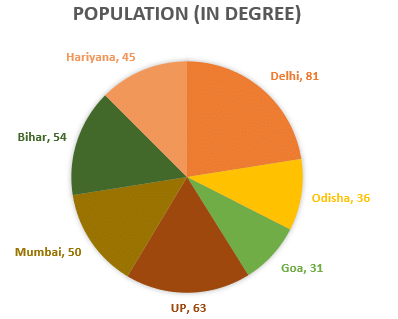How much percent more is the population of UP than that of Odisha?

Detailed Solution for Practice Test: Electronics Engineering (ECE)- 12 - Question 5
Population of UP more than Odisha = 63⁰ - 36⁰ = 27⁰,

Now for percentage we have to calculate how much more population is in UP with respect to Odisha. So,

% more= (27⁰×100)/ 36⁰

= 75%

Practice Test: Electronics Engineering (ECE)- 12 - Question 6

Direction: In the following question, choose the word opposite in meaning to the given word as your answer.

Drought

Detailed Solution for Practice Test: Electronics Engineering (ECE)- 12 - Question 6

Drought (noun) means a prolonged period of abnormally low rainfall, leading to a shortage of water. Its opposite is ‘flood’, which means an overflow of a large amount of water beyond its normal limits.

Practice Test: Electronics Engineering (ECE)- 12 - Question 7

Direction: Study the information carefully and answer the questions given below.

P is standing north of Q at a distance of 6m. R is standing toward east of P at a distance of 6m. R is to the north of S and distance between them is 6m. P is facing north direction, R is facing east, S is facing North and Q is facing towards west direction. All of them walk straight for 3m.

What is the distance between the final position of P and Q?

Detailed Solution for Practice Test: Electronics Engineering (ECE)- 12 - Question 7

3√10m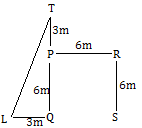Let T be the final position of P and L be the final position of Q.

Now LT = √(32 + 92) = √(9 + 81) = √ 90 = 3√10m

Practice Test: Electronics Engineering (ECE)- 12 - Question 8

In the following question, a statement has been given followed by two arguments numbered I and II. Based on the argument, you have to decide which of the following argument is strong and which is a weak argument.

Statement: Shiva trilogy written by Amish Tripathi is the best fiction book.

Arguments

I. Yes, None other fiction books have three parts.

II. No, the best fiction book is subjective and it always differs from person to person.

Detailed Solution for Practice Test: Electronics Engineering (ECE)- 12 - Question 8

Best fiction book or novel is a subjective thing and differs from person to person. Hence, the second argument is strong.

Practice Test: Electronics Engineering (ECE)- 12 - Question 9

A year after the deadliest Ebola outbreak ever in Africa, the virus has resurfaced in Congo this time around. It is not clear yet how the first victim—a man who has since died—caught the virus. But this outbreak is not the first in Congo; the country has suffered seven prior epidemics of the virus since 1976.

Which of the following is a conclusion that can be drawn from the above paragraph?

Detailed Solution for Practice Test: Electronics Engineering (ECE)- 12 - Question 9

It has been said that the biggest ever Ebola epidemic in Africa struck last year. Hence, (A) is false.

The paragraph says that the first victim of the most recent Ebola outbreak in Congo is dead. Hence, (B) can be concluded from the paragraph.

It has nowhere been mentioned that Congo was not affected by last year’s epidemic. Hence (C) cannot be concluded.

Scientists have not yet figured out how the epidemic resurfaced this time, since the first victim is dead. Hence (D) cannot be concluded.

Practice Test: Electronics Engineering (ECE)- 12 - Question 10

Direction: Following bar graphs shows time taken by different pipes to fill that particular percentage of tank which is described in tabular data. For Ex- Pipe 1 fills the 10 % of tank in 6 mins.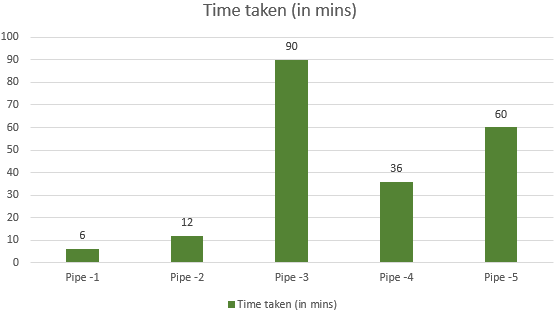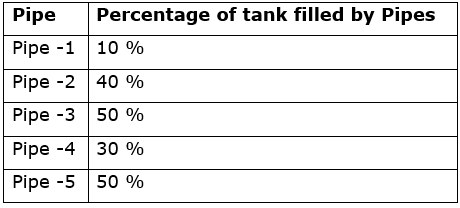A Tank has three pipes; two of them are used to fil the tank while another one used to empty the tank namely: pipe 3, pipe 5 to fill the tank and a new third pipe for making the tank empty. When all three pipes are open, 7/18th part of the tank is filled in 1 hours. What is the ratio of the efficiency of Pipe-3: Pipe 5: all three pipes working together.

Detailed Solution for Practice Test: Electronics Engineering (ECE)- 12 - Question 10

part of work in one hour =

(P3+P5+Third pipE. X 1 = 7/18th part of work

⇒ 7/18th part of work = 1 hr

⇒ Total work done = 18/7 hr by all three pipes

Pipe 3 does 50% of work in 90 mins

100 % of work = 90/50 * 100 = 180 mins = 3 hr

Pipe 5 does 50% of work in 60 mins

100 % of work = 60/50 * 100 = 120 mins = 2 hr

Time Tw Efficiency

Pipe -3 = 3 hr Total work

Pipe-5 = 2 hr Total work

All three = 18/7 hr Total work

hence efficiency ratio: 6: 9: 7

Practice Test: Electronics Engineering (ECE)- 12 - Question 11

Find the unit normal vector of the cone of revolution z2 = 9x2 + 15y2 at a point (1,0,3)

Detailed Solution for Practice Test: Electronics Engineering (ECE)- 12 - Question 11
The unit normal vector is the grad of f = 9x2 + 15y2 - z2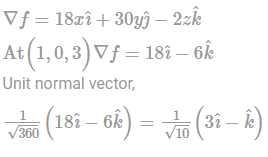Practice Test: Electronics Engineering (ECE)- 12 - Question 12

Minimum of real value function f(x) = (x−1)2/5 occurs at x equal to

Detailed Solution for Practice Test: Electronics Engineering (ECE)- 12 - Question 12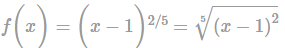as f(x) is square of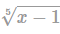hence its min, value be 0 where at (x=1)

Practice Test: Electronics Engineering (ECE)- 12 - Question 13

The transfer function of a system is 10/(s2 + 2s + 10). Find the nature of the given system.

Detailed Solution for Practice Test: Electronics Engineering (ECE)- 12 - Question 13
On comparing the given characteristic equation with the standard second-order transfer function,

ωn2/(s2+2ζωns+ωn2).

Hence, ωn2 = 10 ; 2 ζωn = 2 ⇒ ζ = 0.316

For 0 < ζ < 1, the system is said to be Under-damped.

*Answer can only contain numeric values
Practice Test: Electronics Engineering (ECE)- 12 - Question 14

In a transistor circuit , ICO = ICBO = 2μA , also it is given that α = 0.98

Then the value of ICEO is _______(μA).

Detailed Solution for Practice Test: Electronics Engineering (ECE)- 12 - Question 14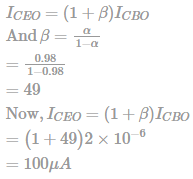Practice Test: Electronics Engineering (ECE)- 12 - Question 15

There are two energy levels E1 and E2. E1 is E eV above the fermi level and E2 is E eV below the fermi level.

P1 = the probability of E1 being occupied by an electron.

P2 = the probability of E2 being empty.

Then, which of the following is correct?

Detailed Solution for Practice Test: Electronics Engineering (ECE)- 12 - Question 15
The Fermi- Dirac probability function also called Fermi function, provides the probability of occupancy of energy levels. It is given by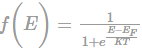where EF is Fermi level or Characteristics energy, for the crystal in eV. The fermi level represents the energy state with 50 percent probability of being filled if no forbidden band exists.

Therefore, P1 And P2 can be calculated by using Fermi- Dirac probability function.

For P1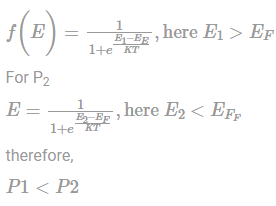Practice Test: Electronics Engineering (ECE)- 12 - Question 16

Match List I (Regions of Bipolar Transistor in a Monolithic IC) with list II (Physical Properties)

List I

a) Emitter

b) Base

c) Collector

d) Substrate

List II

(1) Moderate resistivity

(2) Very high resistivity

(3) Large size

(4) Very high Conductivity

Select the correct answers from the following.

Detailed Solution for Practice Test: Electronics Engineering (ECE)- 12 - Question 16

Emitter – High Doping and very high conductivity

Collector – Large Size

Substrate- Very high resistivity

Base- Moderate resistivity

Practice Test: Electronics Engineering (ECE)- 12 - Question 17

Consider the circuit shown in the figure below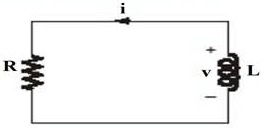v(t) = 80e−1000tV ;t>0

i(t) = 5e−1000tmA ;t>0

the energy dissipated (in μ) by the resistor for 0 < t="" />< 0.6="" ms="" will="" be="" />

Detailed Solution for Practice Test: Electronics Engineering (ECE)- 12 - Question 17
The energy dissipated in the resistance is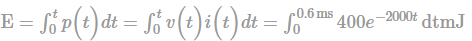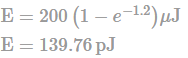Practice Test: Electronics Engineering (ECE)- 12 - Question 18

A practical voltage source consists of:

Detailed Solution for Practice Test: Electronics Engineering (ECE)- 12 - Question 18
An ideal current source is expected to have zero losses, but practically this is not possible. The internal loss of the current source is represented as an internal resistance ‘r’ placed in series with the current source. It is placed in parallel because current remains equal in series components and divides in parallel components.
Practice Test: Electronics Engineering (ECE)- 12 - Question 19

While analysing a two-port network, which of the following parameters are obtained when one-port variables are expressed in terms of the other port variables?

Detailed Solution for Practice Test: Electronics Engineering (ECE)- 12 - Question 19
Expressing one port variables in terms of the other port variables,

(V1,I1) = f(V2, - I2)

The parameters so obtained are the T-parameters or the ABCD-parameters.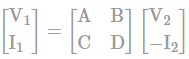These are used for the analysis of the power transmission line. The input and output ports are the sending and the receiving ends respectively.

Practice Test: Electronics Engineering (ECE)- 12 - Question 20

Consider the following sentences regarding a periodic signal

a) A periodic signal always repeats itself after minimum fundamental time period T.

b) A periodic signal is exist from -∞ to +∞ and converse is also true.

c) A periodic signal exist from -∞ to +∞.

Which of the following is/are correct option?

Detailed Solution for Practice Test: Electronics Engineering (ECE)- 12 - Question 20
A- Is the definition of any periodic signal.

B- A periodic signal is only exist from -∞ to +∞ and converse is not true because any signal or anything practically always take birth from origin means from it increases. If it comes from positive to negative then it is a decaying signal not a periodic signal. Thus, option A is the correct answer.

Practice Test: Electronics Engineering (ECE)- 12 - Question 21

The impulse response of a second order under damped system starting from rest is given by

C(t) = 12.5e-8tcos 6t

What are the responsive value of natural frequency and damping ratio of the system?

Detailed Solution for Practice Test: Electronics Engineering (ECE)- 12 - Question 21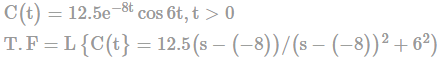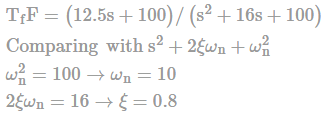Practice Test: Electronics Engineering (ECE)- 12 - Question 22

If x(t) = δ(2t/3), what will be its amplitude at t = 0?

Detailed Solution for Practice Test: Electronics Engineering (ECE)- 12 - Question 22
According to the property of Unit Impulse function

δ(t) = 0, t ≠ 0

≠ 0, t = 0 (Very Large Infinite)

Since delta function represents an infinite amplitude spike at t = 0 having area = 1.

Hence, value of x(t) at t = 0 would be infinite

*Answer can only contain numeric values
Practice Test: Electronics Engineering (ECE)- 12 - Question 23

For the CE amplifier shown, calculate value of lower cutoff frequency FL (in Hz) due to C2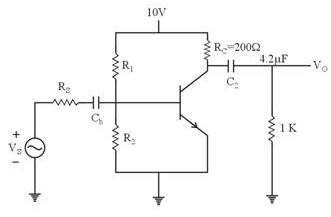Detailed Solution for Practice Test: Electronics Engineering (ECE)- 12 - Question 23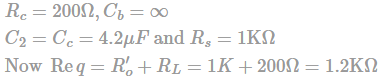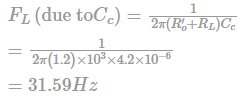Practice Test: Electronics Engineering (ECE)- 12 - Question 24

In the given figure, β = 100, the magnitude of voltage gain (V0/Vin) will be ___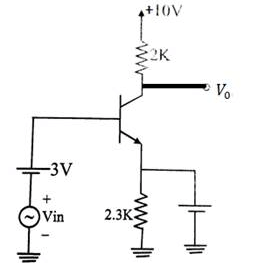Detailed Solution for Practice Test: Electronics Engineering (ECE)- 12 - Question 24
First we draw the AC equivalent of circuit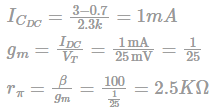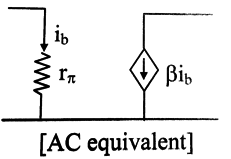Voltage gain (Av) = -gmRc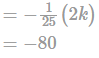Practice Test: Electronics Engineering (ECE)- 12 - Question 25

Calculate the hysteresis width (in Volts) for an inverting Schmitt trigger with feedback fraction 0.5. Assume the supply voltage to be 1

Detailed Solution for Practice Test: Electronics Engineering (ECE)- 12 - Question 25
For a inverting Schmitt trigger.

Given: β = 0.5, Vcc = 10V

Hysteresis Voltage VH = VUT - VLT

VUT = +β x Vcc

VLT = -β x Vcc

VH = 2 x β x Vcc

VH = 2 x 0.5 x 10

VH = 10V

Practice Test: Electronics Engineering (ECE)- 12 - Question 26

For a given sample-and-hold circuit, if the value of the hold capacitor is increased, then

Detailed Solution for Practice Test: Electronics Engineering (ECE)- 12 - Question 26
Capacitar drap rate = dv/dt

For a capacitar,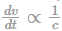Drap rate decreases as capacitar value is increased

For a capacitar,

Q = cv = i × t

⇒ t ∝ c

∴ Acquisition time increases as capacitar value increased

Practice Test: Electronics Engineering (ECE)- 12 - Question 27

The amplitude of a random signal is uniformly distributed between -5 V and 5 V.

If the signal to quantization noise ratio required in uniformly quantizing the signal is 43.5 dB, the step size (in V) of the quantization is approximately

Detailed Solution for Practice Test: Electronics Engineering (ECE)- 12 - Question 27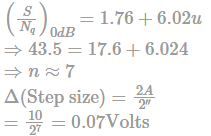Which is close to 0.0667 Volts

Practice Test: Electronics Engineering (ECE)- 12 - Question 28

The Potential (scalar) distribution in free space is given as V = 10y4 + 20x3. if ε0 is permittivity of free space then the charge density ρ at the point (2,0) is

Detailed Solution for Practice Test: Electronics Engineering (ECE)- 12 - Question 28
Here, we have to use Passion’s Equation,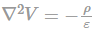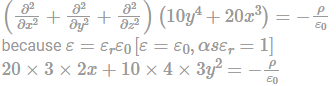At paint (2,0)

ρ = -240ε0

Practice Test: Electronics Engineering (ECE)- 12 - Question 29

For a telephone line, β = 0.02rad/km. If frequency is 1 kHz. Calculate time taken to travel 3.14 km by the wave in

Detailed Solution for Practice Test: Electronics Engineering (ECE)- 12 - Question 29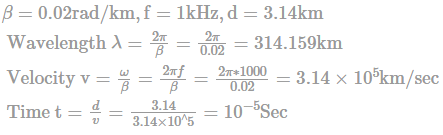Practice Test: Electronics Engineering (ECE)- 12 - Question 30

Consider an air-filled rectangular waveguide with inside dimensions 5 cm x 2cm. If the wave impedance is 222.24 Ω for TM11 mode of propagation. Then the operating frequency (in GHz)is given by

Detailed Solution for Practice Test: Electronics Engineering (ECE)- 12 - Question 30
Given a = 5cm, b 2cm, ηTM11 = 222.24Ω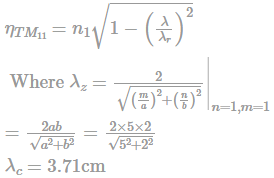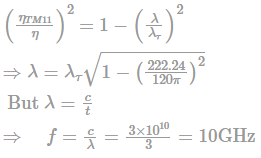*Answer can only contain numeric values
Practice Test: Electronics Engineering (ECE)- 12 - Question 31

A 1MHz uniform plane wave propagates through fresh water for which σ = 0, μr = 1,εr = Calculate wavelength in meters.

Detailed Solution for Practice Test: Electronics Engineering (ECE)- 12 - Question 31
As σ = 0, α will also be 0.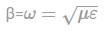but μ = μ0μr and ε = ε0εrAlso Ω = 2π x 106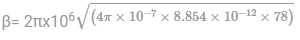λ = 2π/ β = 2π/0.185

λ = 33.963m

Practice Test: Electronics Engineering (ECE)- 12 - Question 32

Which of the following is correct for 1110100 ÷ 1010?

Detailed Solution for Practice Test: Electronics Engineering (ECE)- 12 - Question 32
By simple divide method,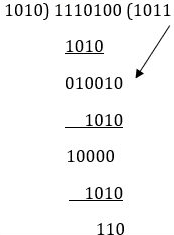Practice Test: Electronics Engineering (ECE)- 12 - Question 33

Which of the following coding scheme is most suitable for coding the successive positions in 8-position digital shaft encoder?

Detailed Solution for Practice Test: Electronics Engineering (ECE)- 12 - Question 33
In digital shaft encoder only one bit change in successive codes, so Gray Code is preferred for digital shaft encoder.

Hence option B is correct as it signify only 1-bit change between two successive codes.

000→001 →011→010→ 110→111→101→111

Practice Test: Electronics Engineering (ECE)- 12 - Question 34

12 signal each band-limited to 5 KHz are to be transmitted over a single channel by freq. division multiplexing. If AM-SSNB modulated guard band of 1 KHz is used, then the bandwidth of the multiplexed signal will be

Detailed Solution for Practice Test: Electronics Engineering (ECE)- 12 - Question 34
The total signal Bankwith = 5 × 12 = 60 KHz

There would be 11 guard band between 12 signal,

So, guard band width

Total band width = 60 + 11 = 71 KHz

Practice Test: Electronics Engineering (ECE)- 12 - Question 35

ln INTEL 8085, suppose the peripheral mapped l/O has address length of M and memory mapped l/O address length of N. Then M+N =_______.

Detailed Solution for Practice Test: Electronics Engineering (ECE)- 12 - Question 35
Peripheral mapped l/O has address length

M = 8

Memory mapped l/O has address length

N = 16

M + N = 8 + 16 = 24

Practice Test: Electronics Engineering (ECE)- 12 - Question 36

Find the bandwidth (in rad/s) of a parallel GLC resonant circuit whose transfer function is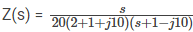Detailed Solution for Practice Test: Electronics Engineering (ECE)- 12 - Question 36
The standard representation of transfer function of a parallel GLC resonant circuit is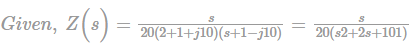On comparing the given T.F with the standard T.F,

Bandwidth = G/C = 2 rad/s.

Practice Test: Electronics Engineering (ECE)- 12 - Question 37

A 70 kHz bandwidth is to accommodate 7 AM broadcast signals simultaneously. The maximum modulation frequency must each station be limited to _____ kHz. Assume that a guard band of 5 kHz is also present.

Detailed Solution for Practice Test: Electronics Engineering (ECE)- 12 - Question 37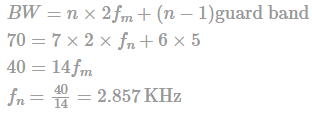Practice Test: Electronics Engineering (ECE)- 12 - Question 38

A carrier frequency modulated with a sinusoidal signal of frequency 4 kHz resulting in a maximum frequency deviation of 7kHz. The bandwidth of the modulated signal is

Detailed Solution for Practice Test: Electronics Engineering (ECE)- 12 - Question 38
Using Carson’s rule

BW = 2(β + 1)fm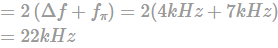Practice Test: Electronics Engineering (ECE)- 12 - Question 39

Consider two real valued signals, x(t) band-limited to [−500 Hz, 500Hz] and y(t) bandlimited to [−1kHz, 1kHz]. For z (t) = x(t). y(t), the Nyquist sampling frequency (in kHz) is __________

Detailed Solution for Practice Test: Electronics Engineering (ECE)- 12 - Question 39

x(t) is band limited to [−500Hz, 500Hz] y(t )is band limited to [−1000Hz, 1000Hz]

z(t) = x (t).y(t)

Multiplication in time domain results convolution in frequency domain.

The range of convolution in frequency domain is [−1500Hz, 1500Hz]

So maximum frequency present in z(t) is 1500Hz Nyquist rate is 3000Hz or 3 kHz

*Answer can only contain numeric values
Practice Test: Electronics Engineering (ECE)- 12 - Question 40

The laplace transform of a signal x(t) is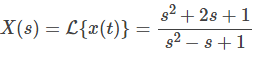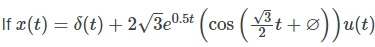then the value of ∅ in degrees is

Detailed Solution for Practice Test: Electronics Engineering (ECE)- 12 - Question 40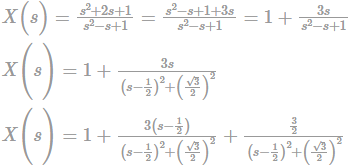Taking inverse laplace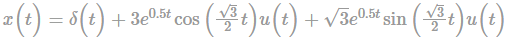We know that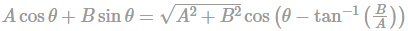Using above equation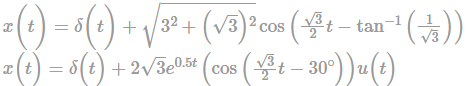So, on comparing θ = -30º

Practice Test: Electronics Engineering (ECE)- 12 - Question 41

A sinusoidal oscillator is built using an amplifier of real gain A, infinite input impedance and the feedback(β) network as shown in the figure.

If Z = jX then Z0 is equal to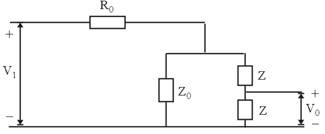Detailed Solution for Practice Test: Electronics Engineering (ECE)- 12 - Question 41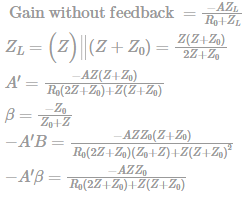For zero phase shift

Z0 + 2 = 0 (As Z0 + 2Z is imaginary)

Z0 = – 2Z = – 2jX

Practice Test: Electronics Engineering (ECE)- 12 - Question 42

Consider the three transistor current source in figure below. Assume all transistor are matched with finite gain and early voltage VA = ∞. The expression for I0 is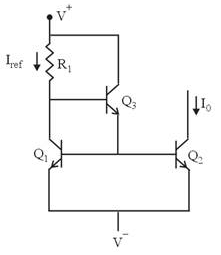Detailed Solution for Practice Test: Electronics Engineering (ECE)- 12 - Question 42

The circuit is as follows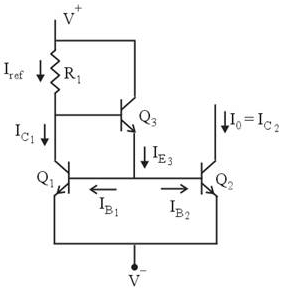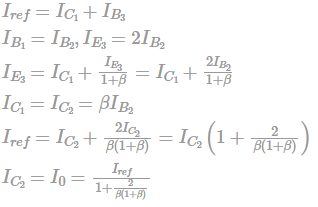Practice Test: Electronics Engineering (ECE)- 12 - Question 43

Two 4-ray signal constellations are shown. It is given that ϕ1 and ϕ2 constitute an orthonormal basis for the two constellations. Assume that the four symbols in both the constellations are equiprobable. Let N0/2 denote the power spectral density of white Gaussian noise.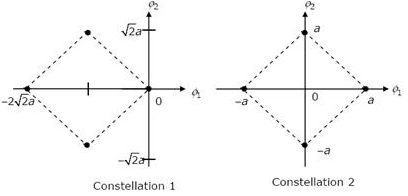The ratio of the average energy of Constellation 1 to the average energy of Constellation 2 is:

Detailed Solution for Practice Test: Electronics Engineering (ECE)- 12 - Question 43

Average energy of constellation 1 is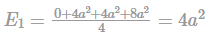Average energy of constellation 2 is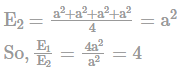Practice Test: Electronics Engineering (ECE)- 12 - Question 44

In an analogue cellular system, find the number of channels when total spectrum allocation (Bt) is 12.5 MHz per simplex band with guard band (Bguard) as 10 kHz having channel bandwidth (Bc) as 30 kHz.

Detailed Solution for Practice Test: Electronics Engineering (ECE)- 12 - Question 44

Number of channels in a FDMA system be calculated as N = Bt-Bguard/Bc

where

N is number of channels

Bt is total spectrum allocation

Bguard is guard band

Bc is channel bandwidth

Calculating channels: N = Bt-Bguard/Bc

N= 12.5 x 106 2(103) / 30 x 103

= 416 channels

Practice Test: Electronics Engineering (ECE)- 12 - Question 45

In synchronous TDM, there are four inputs and data rate of each input connection is 3 kbps. If 1 bit at a time is multiplexed, what is the duration of each frame?

Detailed Solution for Practice Test: Electronics Engineering (ECE)- 12 - Question 45

Given, N = 4n = 1 bit fs = 3kbps

bit raterb = n.N.fs = 1 x 4 x 3kbps = 12 kbps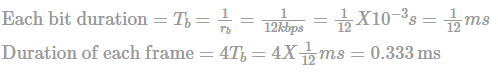*Answer can only contain numeric values
Practice Test: Electronics Engineering (ECE)- 12 - Question 46

A specimen of a Si has a resistivity of 200 Ωk-cm , magnetic flux B = 0.3 Wb/m2 and d = w = 6mm. if the value of hall voltages and hall currents are 60mV and 10 μA. Then the value of μp is ______(cm2/V-sec)

Detailed Solution for Practice Test: Electronics Engineering (ECE)- 12 - Question 46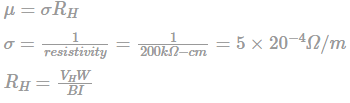= 120 m3/C

Therefore, μ = σRH

=5 × 10-4 × 360

=5 × 10-4 × 120

= 600 cm2/V-sec

Practice Test: Electronics Engineering (ECE)- 12 - Question 47

Silicon is doped with boron to a concentration of 4 × 1017 atoms/cm3. Assuming the intrinsic carrier concentration of silicon to be 1.5×1010/cm3 and the value of kT/q to be 25 mV at 300 K

Compared to undoped silicon, the Fermi level of doped silicon?

Detailed Solution for Practice Test: Electronics Engineering (ECE)- 12 - Question 47

E2 – E1 = kTln (NA/ni)

NA = 4 × 1017

ni = 1.5 × 1010

E2 – E1 = 25 × 10-3 e ln 4 × 1017/1.5×1010 = 0.427eV

Hence Fermi level goes down by 0.427 eV as silicon is doped with boron.

*Answer can only contain numeric values
Practice Test: Electronics Engineering (ECE)- 12 - Question 48

The transmission line shown in figure is 40 m long and has Vg = 15ÃOo Vrms, Zo = 30 + j 60W and VL = 5 Ã–48o Vrms. If the line is matched to the load, then the magnitude of sending – end current Iin is

Detailed Solution for Practice Test: Electronics Engineering (ECE)- 12 - Question 48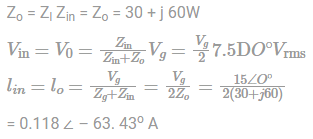Practice Test: Electronics Engineering (ECE)- 12 - Question 49

Consider the following synchronous counter made up of JK, D and T flip-flops.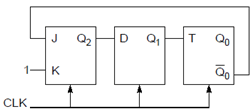Find the modulus value of the counter.

Detailed Solution for Practice Test: Electronics Engineering (ECE)- 12 - Question 49
Consider characteristics equation of J-K flip-flop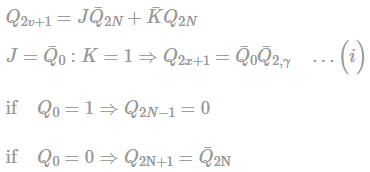Consider characteristic equation of D- Flip-Flop : QN+1 = D

QN+1 = Q2….(ii)

Consider characteristics equation of T-Flip-flop: QN+1 = T⊕QN

Qx+1 = Q1⊕Q0

Using equations (i), (ii) and (iii)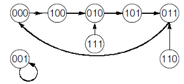The number of used states = 5

∴ Modulus value of the counter = 5.

Practice Test: Electronics Engineering (ECE)- 12 - Question 50

Consider the following k-map, P, Q, R are don’t cares. What should be the values of P, Q, R in order to get the minimized SOP expression?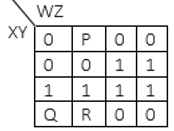Detailed Solution for Practice Test: Electronics Engineering (ECE)- 12 - Question 50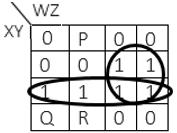SOP = yw + xy = y(x + w) so total is 4 variable

So. P, Q, R are all zero’s.

and if we put the p=0 and Q = 1, R = 1 then we get wy+xy here also 4 variable so and is D correct

Practice Test: Electronics Engineering (ECE)- 12 - Question 51

An antenna consist of 4 identical Hertzian dipoles located along z-axis uniformly with λ/8 space apart.

The group pattern function is_________?

Detailed Solution for Practice Test: Electronics Engineering (ECE)- 12 - Question 51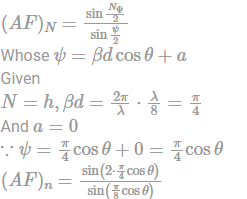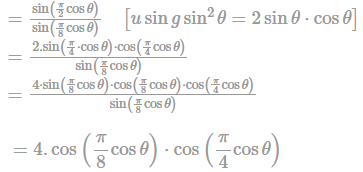Hence option B is correct.

Practice Test: Electronics Engineering (ECE)- 12 - Question 52

The maximum phase lag provided by the given lag compensator is?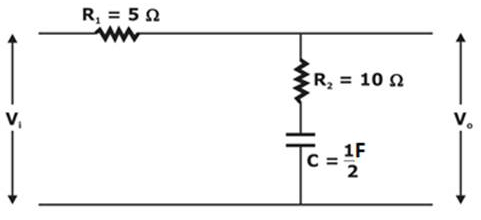Detailed Solution for Practice Test: Electronics Engineering (ECE)- 12 - Question 52

Given network can be expressed as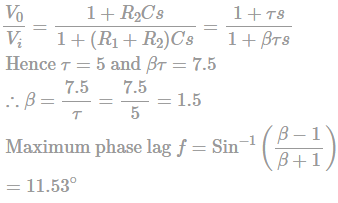Practice Test: Electronics Engineering (ECE)- 12 - Question 53

Find the particular integral of

(D2 – 4D +4) y = e2x sinx cosx

Detailed Solution for Practice Test: Electronics Engineering (ECE)- 12 - Question 53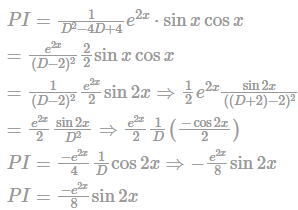Practice Test: Electronics Engineering (ECE)- 12 - Question 54

For a transmission line at frequency of 300 MHz. We have, first maxima occurs at 1/8 m from the load. ZL = jRL , Where RL = k.R0 and R0 = characteristic impedance of line.

Then k = _________? (positive value of k)

Detailed Solution for Practice Test: Electronics Engineering (ECE)- 12 - Question 54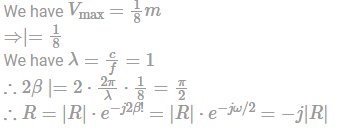Hence, we see that R is purely imaginary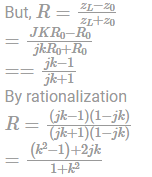As R is purely imaginary

R|real = 0

∴ k2 − 1 = 0

∴ k = ±1

∴ k = 1............positive value

Practice Test: Electronics Engineering (ECE)- 12 - Question 55

A signal m(t) is shown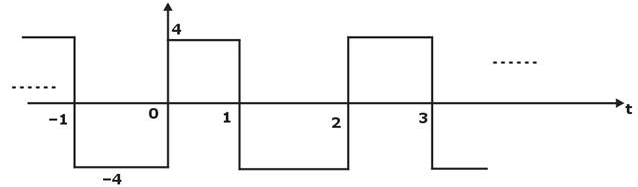This signal is applied to both phase modulator (with kp as phase constant) and frequency modulator (with Kf as frequency constant).

The ratio of kf/kp for same maximum phase deviation is _______?

Detailed Solution for Practice Test: Electronics Engineering (ECE)- 12 - Question 55

In phase modulation maximum phase deviation = kp∣m(t)Imax

Δϕ = 4.kp…(1)

In frequency modulation

Maximum phase deviation = 2πkf∫m(t)dt∣max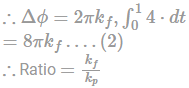Given

The maximum phase deviation for both techniques is same

i.e., from (1) and (2)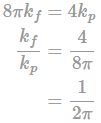Hence option B is correct.

Practice Test: Electronics Engineering (ECE)- 12 - Question 56

Calculate the drift velocity of free electrons in a conductor of cross-section area of 10-4 m2 and current of 400 A is flowing through it, assuming free electron density of conductor to be 9 × 1028 m-3.

Detailed Solution for Practice Test: Electronics Engineering (ECE)- 12 - Question 56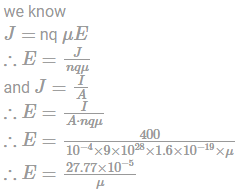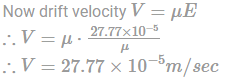Hence option A is correct

Practice Test: Electronics Engineering (ECE)- 12 - Question 57

The inverse z-transform of the signal x(z) = log(1 + z–1) |z| > 1 is?

Detailed Solution for Practice Test: Electronics Engineering (ECE)- 12 - Question 57

Given,

x(z) = log⁡(1 + z − 1)

Differentiating w.r.t z we get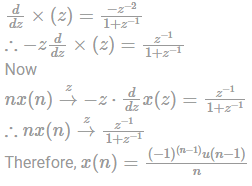Practice Test: Electronics Engineering (ECE)- 12 - Question 58

The input voltage Vi/P is 5 + 4sinωt. Assume diode is ideal. Then the average value of output voltage is?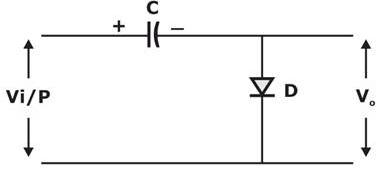Detailed Solution for Practice Test: Electronics Engineering (ECE)- 12 - Question 58

It is a negative clamper circuit and capacitor blocked dc voltage. Hence only Vi/P = 4 sinωt

Hence waveforms are: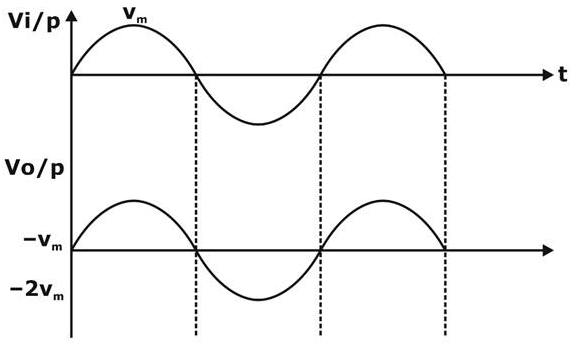Hence avg VO/P = -4 volt

Hence option D is correct.

Practice Test: Electronics Engineering (ECE)- 12 - Question 59

A die is rolled ‘n’ times until 4 appears then the probability that die must rolled more than 5 times before getting a “4”,

Detailed Solution for Practice Test: Electronics Engineering (ECE)- 12 - Question 59

probability of Getting ‘4’ on rolling a die is = ⅙

So, Probability of Not getting ‘4’ is = ⅚

To getting success (get-4), die must be rolled more than 5 times

So,

S

P(x > 5) = 1 − P(x ≤ 5)

Getting success in st trial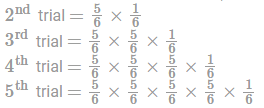So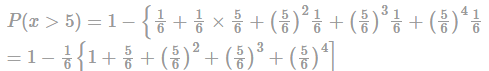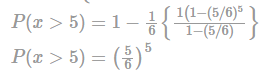*Answer can only contain numeric values
Practice Test: Electronics Engineering (ECE)- 12 - Question 60

For a p-n-p BJT with emitter doping concentration of 1017 / cm3, Base doping of 5 x 1015 / cm3 and collector doping of 3 x 1014 /cm3. Then under no external voltage VCE is ___ volt?

Detailed Solution for Practice Test: Electronics Engineering (ECE)- 12 - Question 60
(0.10, 0.25)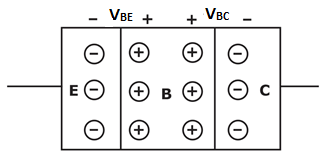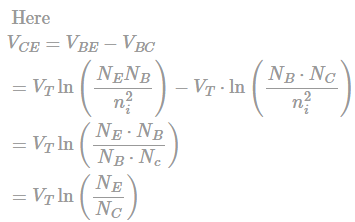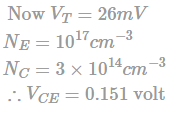*Answer can only contain numeric values
Practice Test: Electronics Engineering (ECE)- 12 - Question 61

The value of the Integral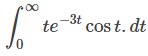is (accurate upto 3 decimal place)

Detailed Solution for Practice Test: Electronics Engineering (ECE)- 12 - Question 61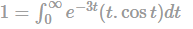The integral is of the form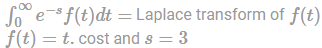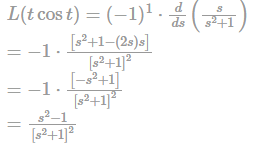Putting the value of s = 3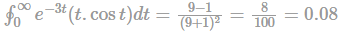*Answer can only contain numeric values
Practice Test: Electronics Engineering (ECE)- 12 - Question 62

The open-loop transfer function unity feedback system is given by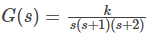The breakaway point of the root locus plot is given by

Detailed Solution for Practice Test: Electronics Engineering (ECE)- 12 - Question 62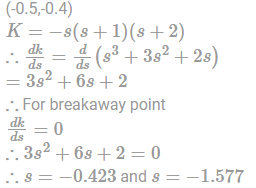But s = −1.577 doesn't lie on the root locus

Hence s = −0.423 is the breakaway point.

*Answer can only contain numeric values
Practice Test: Electronics Engineering (ECE)- 12 - Question 63

An antenna a power input of 50 π watt and an efficiency of 90%.

The radiation intensity has been found to have a 6-maximum value of 140W per solid angle.

The directivity of antenna is ____________.

Detailed Solution for Practice Test: Electronics Engineering (ECE)- 12 - Question 63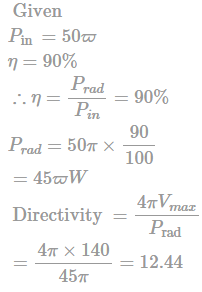*Answer can only contain numeric values
Practice Test: Electronics Engineering (ECE)- 12 - Question 64

Power across 20 Ω resistor is 10 watt. Then the power factor of the coil for the given connection is _____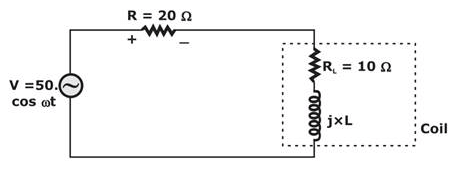Detailed Solution for Practice Test: Electronics Engineering (ECE)- 12 - Question 64
We know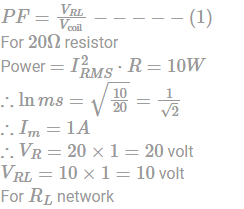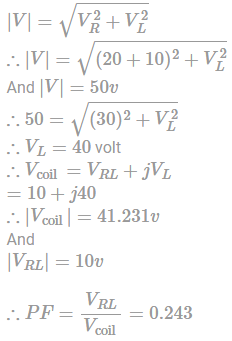Practice Test: Electronics Engineering (ECE)- 12 - Question 65

For given code executed in 8085 μp After the PSW value is?

MVI A, 63H

ORA A

ANI 36 H

DAA

Detailed Solution for Practice Test: Electronics Engineering (ECE)- 12 - Question 65

A = 63

ORA A: A = 63

Flags: P = 1

Z = 0

S = 0

AC = 0

C = 0

ANI: 63 AND 36

= 22 (0010 0010)

Flags: P = 1

Z = 0

S = 0

AC = 1

C = 0

DAA:

as AC = 1 add 6 (0110) to lower nibble

As C = 0 do not add 6 to upper nibble.

∴ ACC ⇒ 0010 0010

+ 0000 0110

0010 1000

= 28 H

Flags: cy = 0

P = 1

AC = 0

Z = 0

S = 0

## GATE ECE (Electronics) 2024 Mock Test Series

22 docs|263 tests
Information about Practice Test: Electronics Engineering (ECE)- 12 Page
In this test you can find the Exam questions for Practice Test: Electronics Engineering (ECE)- 12 solved & explained in the simplest way possible. Besides giving Questions and answers for Practice Test: Electronics Engineering (ECE)- 12, EduRev gives you an ample number of Online tests for practice

## GATE ECE (Electronics) 2024 Mock Test Series

22 docs|263 tests